Test: Basic Trigonometric Formulas

# Test: Basic Trigonometric Formulas - Commerce

Test Description

## 10 Questions MCQ Test Mathematics (Maths) Class 11 - Test: Basic Trigonometric Formulas

Test: Basic Trigonometric Formulas for Commerce 2023 is part of Mathematics (Maths) Class 11 preparation. The Test: Basic Trigonometric Formulas questions and answers have been prepared according to the Commerce exam syllabus.The Test: Basic Trigonometric Formulas MCQs are made for Commerce 2023 Exam. Find important definitions, questions, notes, meanings, examples, exercises, MCQs and online tests for Test: Basic Trigonometric Formulas below.
Solutions of Test: Basic Trigonometric Formulas questions in English are available as part of our Mathematics (Maths) Class 11 for Commerce & Test: Basic Trigonometric Formulas solutions in Hindi for Mathematics (Maths) Class 11 course. Download more important topics, notes, lectures and mock test series for Commerce Exam by signing up for free. Attempt Test: Basic Trigonometric Formulas | 10 questions in 10 minutes | Mock test for Commerce preparation | Free important questions MCQ to study Mathematics (Maths) Class 11 for Commerce Exam | Download free PDF with solutions
 1 Crore+ students have signed up on EduRev. Have you?
Test: Basic Trigonometric Formulas - Question 1

### What is the value of cos x in second quadrant if sin x = 3/5 in II quadrant

Detailed Solution for Test: Basic Trigonometric Formulas - Question 1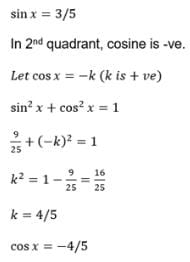Test: Basic Trigonometric Formulas - Question 2

### What is the value of cos 41π/4

Detailed Solution for Test: Basic Trigonometric Formulas - Question 2

Step-by-step explanation: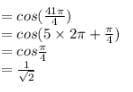Test: Basic Trigonometric Formulas - Question 3

### In which quadrant are sin, cos and tan positive?

Detailed Solution for Test: Basic Trigonometric Formulas - Question 3
• All three of them are positive in Quadrant I
• Sine only is positive in Quadrant II
• Tangent only is positive in Quadrant III
• Cosine only is positive in Quadrant IV
•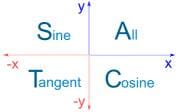Test: Basic Trigonometric Formulas - Question 4

What is the range of cos function?

Detailed Solution for Test: Basic Trigonometric Formulas - Question 4

Just look at the graph of cosine.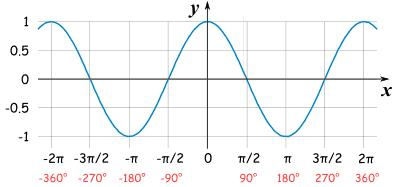We know , Range of a function is the set of all possible outputs for that function. If you look at any 2π interval, the cosine function is periodic after every 2π.  So the range for cos function is [-1,1]

Test: Basic Trigonometric Formulas - Question 5

What is the value of sin 7π ?

Detailed Solution for Test: Basic Trigonometric Formulas - Question 5

Sin 7π = Sin 7*180 = Sin 2π * 7  = 0

Test: Basic Trigonometric Formulas - Question 6

Which of the following cannot be the value of cos θ.

Detailed Solution for Test: Basic Trigonometric Formulas - Question 6

√2 cannot be the value for Cosθ.

The values of  Cos θ at different angles are given below :

Cos0°=1

Cos30°=√3/2

Cos45°=1/√2

Cos60°=1/2

Cos90°=0

Test: Basic Trigonometric Formulas - Question 7

What is the sign of the sinA and tanA in third quadrant respectively

Detailed Solution for Test: Basic Trigonometric Formulas - Question 7

The sign of the sinA and tanA in third quadrant is negative and positive.

Test: Basic Trigonometric Formulas - Question 8

tan x = - 5/12, x lies in the second quadrant. So sinx = ?

Detailed Solution for Test: Basic Trigonometric Formulas - Question 8

tanx = -5/12
Therefore perpendicular = -5, base = 12
Applying pythagoras theorem,
(hyp)2 = (per)2 + (base)2
⇒ (-5)2 + (12)2
hyp = [25+144]1/2
hyp = (169)1/2
hyp = 13
sinx = perpendicular/hypotenous
= -5/13
In second quadrant, only sin x, cosec x are positive
So. sinx = 5/13

Test: Basic Trigonometric Formulas - Question 9

What is the sign of the sec θ and cosec θ in second quadrant respectively?

Detailed Solution for Test: Basic Trigonometric Formulas - Question 9

In quadrant sin, cos tan, cot, sec, cosec all +ve .In second quadrant sin and cosec are +ve. in 3rd quadrant tan and cot are positive.And in 4th cos and sec are +ve.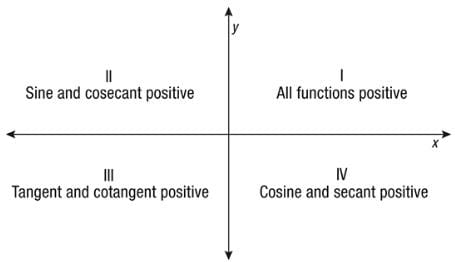Test: Basic Trigonometric Formulas - Question 10

Identify the odd one out from the following

## Mathematics (Maths) Class 11

157 videos|210 docs|132 tests
Information about Test: Basic Trigonometric Formulas Page
In this test you can find the Exam questions for Test: Basic Trigonometric Formulas solved & explained in the simplest way possible. Besides giving Questions and answers for Test: Basic Trigonometric Formulas, EduRev gives you an ample number of Online tests for practice

## Mathematics (Maths) Class 11

157 videos|210 docs|132 tests(Scan QR code)